### Home > CAAC > Chapter 10 > Lesson 10.2.1 > Problem10-52

10-52.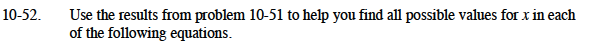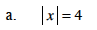The absolute values of what two numbers equals 4?

x = −4 or 4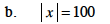Follow the steps of part (a).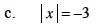Can the absolute value of any number be negative?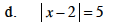The absolute values of −5 and 5 equal 5.

x − 2 = 5 or x − 2 = −5
Now solve both equations for x.

x = 7 or −3